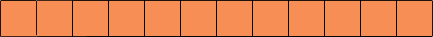Name: Catherine Who is asking: Parent Level of the question: Secondary Question: My daughter needs to solve this, and I can't help. using 12 squares, make a number of patterns (squares joined). Find the perimeters. Find out how many points in the shape have four radiating lines i.e. are two lines intersecting. Write an equation to state the relationship between the lines and the perimeter. Hi Catherine, I would suggest that you experiment with something physical. I used tea bags. By "squares joined" I assumed that two squares had to have adjacent sides not just vertices. That is you can havebut notThe simplest pattern is probably the squares all in a lineIf you assume that each squarer has a side of length 1 then this has a perimeter of 26. So does my first diagram and so doesbuthas a perimeter of 24. I am going to draw the last two diagrams again and mark the points that have "four radiating lines".I have marked these points with two colours, red if the point is completely surrounded by the orange squares and black otherwise. Try some different arrangements, measure the perimeter, count the points of each kind that have "four radiating lines" and see if you can find a relationship among these three numbers. Penny